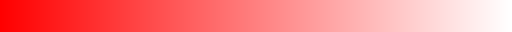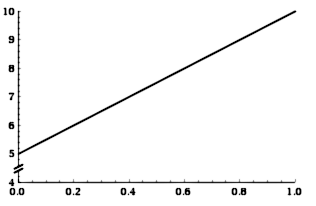# Color Interpolation

The easiest form of interpolation is linear interpolation by using the function $$\text{linpol}(p,a,b) := a \cdot (1 - p) + b \cdot p$$ where $$a$$ is 'from' and $$b$$ is to and $$0.0 \leq p \leq 1.0$$, meaning if $$p = 0.0$$ then $$\text{linpol}(p,a,b) = a$$ and if $$p = 1.0$$ then $$\text{linpol}(p,a,b) = b$$. If we want to interpolate RGB colors we interpolate each channel seperately:

$$\text{linpol}(p,\{r_{0},g_{0},b_{0}\},\{r_{1},g_{1},b_{1}\} := \{\\r_{0} \cdot (1 - p) + r_{1} \cdot p, \\ g_{0} \cdot (1 - p) + g_{1} \cdot p, \\ b_{0} \cdot (1 - p) + b_{1} \cdot p\\\}$$

If we want to interpolate between more colors we do it in steps. Interpolating between color 1 and color 2, color 2 and color 3, ... and so forth. We divide $$0..1$$ into intervals of size $$s$$ and calculate in which interval $$p$$ falls into and then interpolate between the corresponding colors. For example with three colors we interpolate between color 1 and color 2 for $$0.0 \leq p \leq 0.5$$ and between color 2 and color 3 for $$0.5 \leq p \leq 1.0$$. With this knowledge we can create color gradients.1st image: Gradient from red to white. 2nd image: Gradient using heatmap colors.Plot for $$\text{linpol}(x, 5, 10)$$ for $$0.0 \leq x \leq 1.0$$.

## The algorithm

 proc linpol(f64 p, color a, color b) color begin color c := (0.0, 0.0, 0.0) c = a * (1 - p) + b * p c = a * (1 - p) + b * p c = a * (1 - p) + b * p return c end proc linpol_n(f64 p, color[] colors) color begin f64 step_size := 1.0 / f64(size(colors) - 1) u32 color_index := u32(floor(p / step_size)) ; shift p to 0..step_size f64 pi = p - (step_size * f64(color_index)) ; scale 0..step_size to 0..1 pi /= step_size ; color for pi ~= 0.0 color a := colors[color_index] ; color for pi ~= 1.0 ; in the edge case of p = 1.0 color_index points to the last color ; so color_index+1 would be invalid. color b := colors[min(size(colors)-1, color_index+1)] return linpol(pi, a, b) end